# Test: Floating Point Number- 2

## 12 Questions MCQ Test GATE Computer Science Engineering(CSE) 2023 Mock Test Series | Test: Floating Point Number- 2

Description
Attempt Test: Floating Point Number- 2 | 12 questions in 45 minutes | Mock test for Computer Science Engineering (CSE) preparation | Free important questions MCQ to study GATE Computer Science Engineering(CSE) 2023 Mock Test Series for Computer Science Engineering (CSE) Exam | Download free PDF with solutions
QUESTION: 1

### For interval arithmetic best rounding technique use is ________.

Solution:

Rounding to plus and minus infinity are useful in implementing a technique known as interval arithmetic. Interval arithmetic provides an efficient method for monitoring and controlling errors in floating point computations by producing two values for each result. The two values correspond to the lower and upper endpoints of an interval that contains the true result. The width of the interval, which is the difference between the upper and lower endpoints, indicates the accuracy of the result of the endpoints of an interval are not representable then the interval endpoints are rounded down and up respectively.

QUESTION: 2

### When multiplicand Y is multiplied by multiplier X = xn-1xn-2 ... x0 using bit- pair recording in Booth’s algorithm, partial products are generated according to the following table: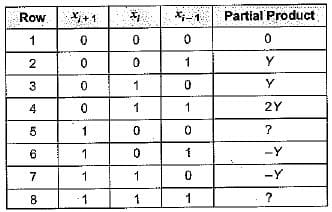Q. The partial products for rows 5 and 8 are

Solution: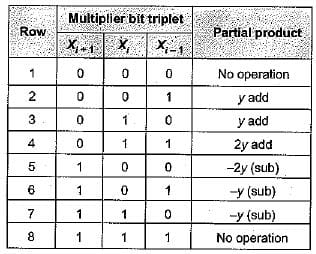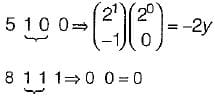QUESTION: 3

### Consider the following floating point format: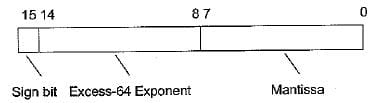Mantissa is a pure fraction is sign-magnitude form. Q. The decimal number 0.239 x 213 has the following hexadecimal representation without normalization and rounding off

Solution:

The decimal number is 0.239 x 213
We have to find hexadecimal representation without normalization.
Biased exponent = 13 + 64 = 77
Representing 77 in binary
(77)10 = (1001101)2
Representing mantissa in binary

(0.239)10 = 0.00111101000101
Floating point representation is as follows: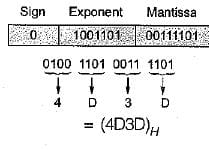QUESTION: 4

Consider the following floating point format:Mantissa is a pure fraction is sign-magnitude form.

Q. The normalized representation for the above format is specified as follows. The mantissa has an implicit 1 preceding the binary (radix) point. Assume that only 0’s are padded in while shifting a field. The normalized representation of the above number (0.239 x 213) is

Solution:

The decimal number is 0.239 x 213
We have to find hexadecimal representation with normalization.
Binary representation of 0.239 is 0.00111101000101
∴ N is (0.00111101000101) x 213
After normalization we have 1.11101000101 x 210
Then, biased exponent - 10 + 64 = 74 Representating biased exponent 74 in binary (74)10 = (1001010)2
• Floating point representation of number is as follows-: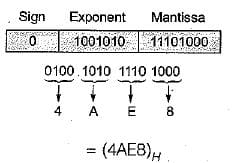QUESTION: 5

The addition of 4-bit, two’s complement, binary numbers 1101 and 0100 results in

Solution: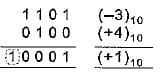No overflow

QUESTION: 6

(C012.25)H -(10111001110.101)B =

Solution: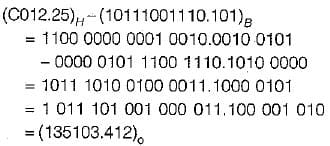Binary subtraction is like decimal subtraction:
0 - 0 = 0, 1 - 1 = 0, 1 - 0 = 1,
0 - 1 = 1 with 1 borrow.

QUESTION: 7

The following bit pattern represents a floating point number in IEEE 754 single precision format: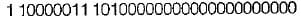The value of the number in decimal form is

Solution:

Sign bit is 1 implies number is negative. Exponent bits: 10000011 Exponent is added with 127 bias in IEEE single precision format.
So, Actual exponent
= 10000011 - 127
= 131 - 127 = 4
Mantissa bits: 101000000000000000000000
In IEEE format, an implied 1 is before mantissa.
Hence the Number is -1.101*24
= - (11010)2 = - 26

QUESTION: 8

In the IEEE floating point representation the hexadecimal value 0x00000000 corresponds to

Solution:

An exponent of 0 together with a fraction of 0 with positive sign represents +0 which is a special value.

QUESTION: 9

The two numbers given below are multiplied using the Booth’s algorithm.
Multiplicand: 0101101011101110
Multiplier: 0111011110111101

Q. How many additions/Subtractions are required for the multiplication of the above two numbers?

Solution:

Apply Booth’s algorithm: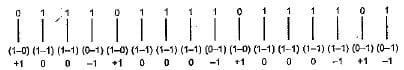4 additions and 4 substractions total 8 operations.

QUESTION: 10

P is a 16-bit signed integer. The 2’s complement representation of P is (F87B)16. The 2's complement representation of 8*P is

Solution:

2’s complement of P is given as (F87B)16
2’s complement of 8 x P = 23 x (F87B)16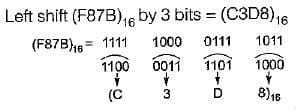QUESTION: 11

The smallest integer that can be represent by and 8-bit number in 2’s complement form is

Solution:

Range of 2’s complement no is
(-2n-1 to 2n-1 - 1)
So minimum no represents by 8 bit is
- 28 - 1 = - 128
maximum no represented by 8 bit is
28 - 1 - 1 = 127

QUESTION: 12

The n-bit fixed-point representation of an unsigned real number X uses f bits for the fraction part. Let i = n - f. The range of decimal values for X in this representation is

Solution:

Let n = 5 bit
f = 2 bit
So i = 5 - 2 = 3 bit
Min value : 000.00
⇒ 0
Max value : 111.11
⇒ 7.75
[2i - 2-f]
⇒ [23 - 2-2]
⇒ 8 - 0.25 = 7.75Use Code STAYHOME200 and get INR 200 additional OFF Use Coupon Code